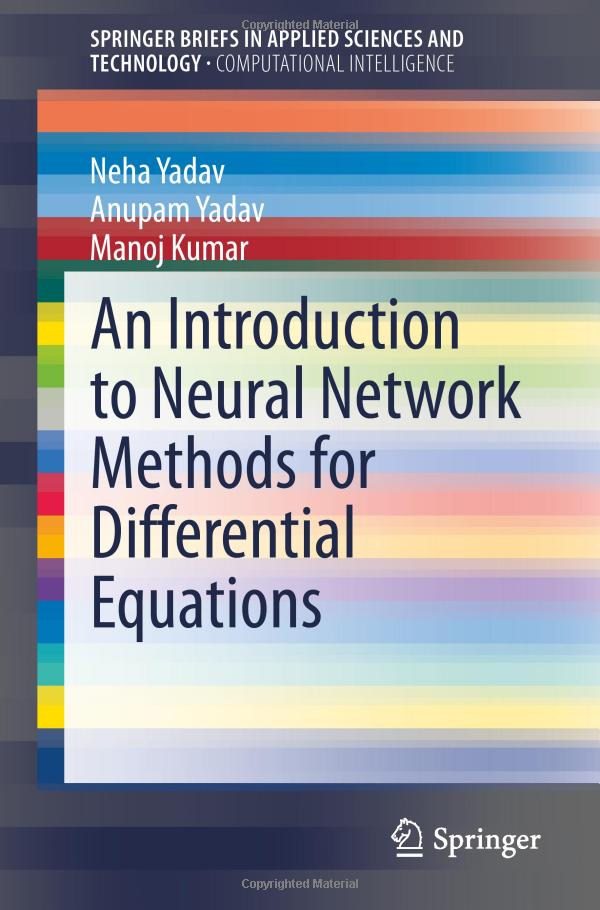# An Introduction To Neural Network Methods For Differential Equations by Neha Yadav / 2015 / English / PDF

This book introduces a variety of neural network methods for solving differential equations arising in science and engineering. The emphasis is placed on a deep understanding of the neural network techniques, which has been presented in a mostly heuristic and intuitive manner. This approach will enable the reader to understand the working, efficiency and shortcomings of each neural network technique for solving differential equations. The objective of this book is to provide the reader with a sound understanding of the foundations of neural networks and a comprehensive introduction to neural network methods for solving differential equations together with recent developments in the techniques and their applications. The book comprises four major sections. Section I consists of a brief overview of differential equations and the relevant physical problems arising in science and engineering. Section II illustrates the history of neural networks starting from their beginnings in the 1940s through to the renewed interest of the 1980s. A general introduction to neural networks and learning technologies is presented in Section III. This section also includes the description of the multilayer perceptron and its learning methods. In Section IV, the different neural network methods for solving differential equations are introduced, including discussion of the most recent developments in the field. Advanced students and researchers in mathematics, computer science and various disciplines in science and engineering will find this book a valuable reference source.

views: 876